## How to Calculate and Solve for Quantity of Heat Loss | Fuel and Furnaces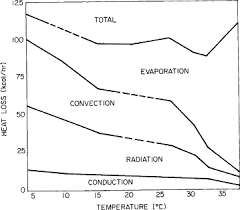The image above represents quantity of heat loss.

To compute for quantity of heat loss, four essential parameters are needed and these parameters are Initial Temperature (T1), Final Temperature (T2), a and E.

The formula for calculating quantity of heat loss:

Q = a(T1 – T2)5/4 + 4.88E[(T1 + 273/100)4 – (T2 + 273/100)4]

Where:

Q = Quantity of Heat Loss
T1 = Initial Temperature
T2 = Final Temperature

Let’s solve an example;
Find the quantity of heat loss when the initial temperature is 21, the final temperature is 17, a is 14 and E is 15.

This implies that;

T1 = Initial Temperature = 21
T2 = Final Temperature = 17
a = 14
E = 15

Q = a(T1 – T2)5/4 + 4.88E[(T1 + 273/100)4 – (T2 + 273/100)4]
Q = 14(21 – 17)5/4 + 4.88(15)[(21 + 273/100)4 – (17 + 273/100)4]
Q = 14(4)5/4 + 4.88(15)[74.71 – 70.72809]
Q = 14(5.65) + 4.88(15)(3.98)
Q = 79.19 + 291.608
Q = 370.80

Therefore, the quantity of heat loss is 370.80 J/Kg K.

## How to Calculate and Solve for Total Heat Loss in Furnace | Fuel and Furnaces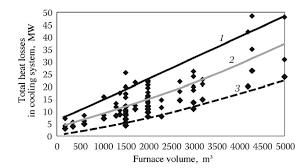The image above represents total heat loss in furnace.

To compute for total heat loss in furnace, four essential parameters are needed and these parameters are Absolute Temperature (Kelvin) (T), Factor of Total Radiation (a), Area of Opening (A) and Time (Hours) (H).

The formula for calculating total heat loss in furnace:

Q = 4.88(T/100)4 x a x AH

Where:

Q = Total Heat Loss in Furnace
T = Absolute Temperature (Kelvin)
a = Factor of Total Radiation
A = Area of Opening
H = Time (Hours)

Let’s solve an example;
Find the total heat loss in furnace when the absolute temperature is 22, the factor of total radiation is 14, the area of opening is 10 and the time is 18.

This implies that;

T = Absolute Temperature (Kelvin) = 22
a = Factor of Total Radiation = 14
A = Area of Opening = 10
H = Time (Hours) = 18

Q = 4.88(T/100)4 x a x AH
Q = 4.88(22/100)4 x 14 x (10)(18)
Q = 4.88(0.22)4 x 14 x 180
Q = 4.88(0.00234256) x 2520
Q = 28.807

Therefore, the total heat loss in furnace is 28.807 J/Kg K.

## How to Calculate and Solve for %Heat Loss through Body | Fuel and Furnaces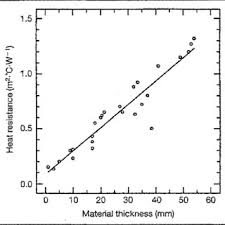The image above represents %heat loss through body.

To compute for %heat loss through body, four essential parameters are needed and these parameters are Heat Loss i (Hi), Heat Loss ∂i (H∂i), Gross Calorific Value of Oil (GCVoil) and Quantity of Oil per Hour (QH).

The formula for calculating %heat loss through body:

%QL = 100(Hi + H∂i/GCVoil + QH)

Where:

%QL = %Heat Loss through Body
Hi = Heat Loss i
H∂i = Heat Loss ∂i
GCVoil = Gross Calorific Value of Oil
QH = Quantity of Oil per Hour

Let’s solve an example;
Find the %heat loss through body when the heat loss is 21, the heat loss is 14, the gross calorific value of oil is 10 and the quantity of oil per hour is 11.

This implies that;

Hi = Heat Loss i = 21
H∂i = Heat Loss ∂i = 14
GCVoil = Gross Calorific Value of Oil = 10
QH = Quantity of Oil per Hour = 11

%QL = 100(Hi + H∂i/GCVoil + QH)
%QL = 100(21 + 14/10 + 11)
%QL = 100(35/21)
%QL = 100(1.66)
%QL = 166.66

Therefore, the %heat loss through body is 166.66%

## How to Calculate and Solve for %Heat Loss through Furnace Skin | Fuel and Furnaces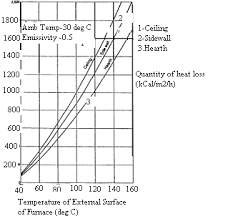The image above represents %heat loss through furnace skin.

To compute for %heat loss through furnace skin, two essential parameters are needed and these parameters are Heat Loss from Roof and Walls (HRW) and Area of Roof and Walls (ARW).

The formula for calculating %heat loss through furnace skin:

%QL = 100(HRW/ARW)

Where:

%QL = %Heat Loss through Furnace Skin (Roof)
HRW = Heat Loss from Roof and Walls
ARW = Area of Roof and Walls

Let’s solve an example;
Find the %heat loss through furnace skin when the heat loss from roof and walls is 15 and the area of roof and walls is 3.

This implies that;

HRW = Heat Loss from Roof and Walls = 15
ARW = Area of Roof and Walls = 3

%QL = 100(HRW/ARW)
%QL = 100(15/3)
%QL = 100(5)
%QL = 500

Therefore, the %heat loss through furnace skin is 500%.

Calculating the Heat Loss from Roof and Walls when the %Heat Loss through Furnace Skin and the Area of Roof and Walls is Given.

HRW = %QL x ARW / 100

Where;

HRW = Heat Loss from Roof and Walls
%QL = %Heat Loss through Furnace Skin (Roof)
ARW = Area of Roof and Walls

Let’s solve an example;
Find the heat loss from roof and walls when the %heat loss through furnace skin is 20 and the area of roof and walls is 12.

This implies that;

%QL = %Heat Loss through Furnace Skin (Roof) = 20
ARW = Area of Roof and Walls = 12

HRW = %QL x ARW / 100
HRW = 20 x 12 / 100
HRW = 240 / 100
HRW = 2.4

Therefore, the heat loss from roof and wall is 2.4%.

## How to Calculate and Solve for %Heat Loss from Openings in Furnace | Fuel and Furnaces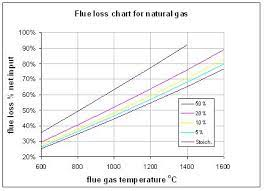The image above represents %heat loss from openings in furnace.

To compute for %heat loss from openings in furnace, five essential parameters are needed and these parameters are Black Body Radiation (BR), Emissivity (E), Radiation Factor (RF), Quantity of Oil (Q) and Gross Calorific Value of Oil (GCVoil).

The formula for calculating %heat loss from openings in furnace:

%QL = 100(BR x E x RF/Q x GCVoil)

Where:

%QL = %Heat Loss from Openings in Furnace
E = Emissivity
Q = Quantity of Oil
GCVoil = Gross Calorific Value of Oil

Let’s solve an example;
Find the %heat loss from openings in furnace when the black body radiation is 15, the emissivity is 6, the radiation factor is 3, the quantity of oil is 24 and the gross calorific value of oil is 9.

This implies that;

BR = Black Body Radiation = 15
E = Emissivity = 6
RF = Radiation Factor = 3
Q = Quantity of Oil = 24
GCVoil = Gross Calorific Value of Oil = 9

%QL = 100(BR x E x RF/Q x GCVoil)
%QL = 100(15 x 6 x 3/24 x 9)
%QL = 100(270/216)
%QL = 100(1.25)
%QL = 125

Therefore, the %heat loss from openings in furnace is 125%.

## How to Calculate and Solve for %Heat Loss due to Hydrogen in Fuel | Fuel and Furnaces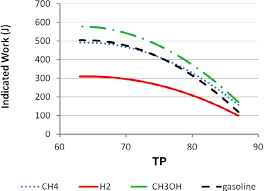The image above represents %heat loss due to hydrogen in fuel.

To compute for %heat loss due to hydrogen in fuel, five essential parameters are needed and these parameters are Mass of Hydrogen (H2), Specific Heat (Cp), Flue Gas Temperature (Tfg), Ambient Temperature (Tamb) and Gross Calorific Value of Fuel (GCVf).

The formula for calculating %heat loss due to hydrogen in fuel:

%QL = 100(9H2(584 + Cp(Tfg – Tamb))GCVf)

Where:

%QL = %Heat Loss due to Hydrogen in Fuel
H2 = Mass of Hydrogen
Cp = Specific Heat
Tfg = Flue Gas Temperature
Tamb = Ambient Temperature
GCVf = Gross Calorific Value of Fuel

Let’s solve an example;
Find the %heat loss due to hydrogen in fuel when the mass of hydrogen is 21, the specific heat is 13, the flue gas temperature is 11, the ambient temperature is 16 and the gross calorific value of fuel is 14.

This implies that;

H2 = Mass of Hydrogen = 21
Cp = Specific Heat = 13
Tfg = Flue Gas Temperature = 11
Tamb = Ambient Temperature = 16
GCVf = Gross Calorific Value of Fuel = 14

%QL = 100(9H2(584 + Cp(Tfg – Tamb))GCVf)
%QL = 100(9(21)(584 + 13(11 – 16))/14)
%QL = 100((189)(584 + 13(-5))/14)
%QL = 100((189)(584 + (-65))/14)
%QL = 100((189)(519)/14)
%QL = 100((98091)/14)
%QL = 100(7006.5)
%QL = 700650

Therefore, the %heat loss due to hydrogen fuel is 700650%.

## How to Calculate and Solve for %Heat Loss from Moisture in Fuel | Fuel and Furnaces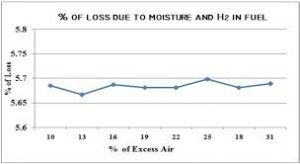The image above represents %heat loss from moisture in fuel.

To compute for %heat loss from moisture in fuel, five essential parameters are needed and these parameters are Mass of the Moisture in Fuel (m), Specific Heat of Stock (Cp), Flue Gas Temperature (Tfg), Ambient Temperature (Tamb) and Gross Calorific Value of Fuel (GCVf).

The formula for calculating %heat loss from moisture in fuel:

%QL = 100(m(584 + Cp(Tfg – Tamb))/GCVf)

Where:

%QL = %Heat Loss from Moisture in Fuel
m = Mass of the Moisture in Fuel
Cp = Specific Heat of Stock
Tfg = Flue Gas Temperature
Tamb = Ambient Temperature
GCVf = Gross Calorific Value of Fuel

Let’s solve an example;
Find the %heat loss from moisture in fuel when the mass of the moisture in fuel is 8, the specific heat of stock is 4, the flue gas temperature is 2, the ambient temperature is 10 and the gross calorific value of fuel is 6.

This implies that;

m = Mass of the Moisture in Fuel = 8
Cp = Specific Heat of Stock = 4
Tfg = Flue Gas Temperature = 2
Tamb = Ambient Temperature = 10
GCVf = Gross Calorific Value of Fuel = 6

%QL = 100(m(584 + Cp(Tfg – Tamb))/GCVf)
%QL = 100(8(584 + 4(2 – 10))/6)
%QL = 100(8(584 + 4(-8))/6)
%QL = 100(8(584 + -32)/6)
%QL = 100(8(552)/6)
%QL = 100(4416/6)
%QL = 100(736)
%QL = 73600

Therefore, the %heat loss from moisture in fuel is 73600%.

## How to Calculate and Solve for %Heat Loss in Flue Gas | Fuel and Furnaces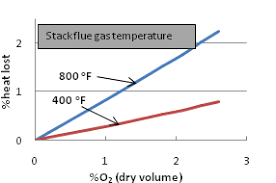The image above represents %heat loss in flue gas.

To compute for %heat loss in flue gas, four essential parameters are needed and these parameters are Mass (m), Specific Heat of Stock (Cp), Change in Temperature (ΔT) and Gross Calorific Value of Fuel (GCVf).

The formula for calculating %heat loss in fuel gas:

%QL = 100(mCpΔT/GCVf)

Where:

%QL = %Heat Loss in Flue Gas
m = Mass
Cp = Specific Heat of Stock
ΔT = Change in Temperature
GCVf = Gross Calorific Value of Fuel

Let’s solve an example;
Find the %heat loss in flue gas when the mass is 10, the specific heat of shock is 14, the change in temperature is 12 and the gross calorific value of fuel is 18.

This implies that;

m = Mass = 10
Cp = Specific Heat of Stock = 14
ΔT = Change in Temperature = 12
GCVf = Gross Calorific Value of Fuel = 18

%QL = 100(mCpΔT/GCVf)
%QL = 100(10(14)(12)/18)
%QL = 100(1680/18)
%QL = 100(93.33)
%QL = 9333.3

Therefore, the %heat loss of flue gas is 9333.3%.

## How to Calculate and Solve for Quantity of Heat | Fuel and Furnaces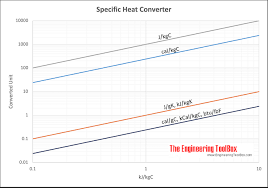The image above represents quantity of heat.

To compute for quantity of heat, three essential parameters are needed and these parameters are Mass of the Stock (m), Specific Heat of Stock (Cp) and Change in Temperature (ΔT).

The formula for calculating quantity of heat:

Q = mCpΔT

Where:

Q = Quantity of Heat
m = Mass of the Stock
Cp = Specific Heat of Stock
ΔT = Change in Temperature

Let’s solve an example;
Find the quantity of heat when the mass of the stock is 18, the specific heat of stock is 10 and the change in temperature is 12.

This implies that;

m = Mass of the Stock = 18
Cp = Specific Heat of Stock = 10
ΔT = Change in Temperature = 12

Q = mCpΔT
Q = 18(10)(12)
Q = 2160

Therefore, the quantity of heat is 2160 J/kg K.

Calculating the Mass of the Stock when the Quantity of Heat, the Specific Heat of Stock and the Change in Temperature is Given.

m = Q / Cp x ΔT

Where;

m = Mass of the Stock
Q = Quantity of Heat
Cp = Specific Heat of Stock
ΔT = Change in Temperature

Let’s solve an example;
Find the mass of the stock when the quantity of heat is 15, the specific heat of stock is 3 and the change in temperature is 4.

This implies that;

Q = Quantity of Heat = 15
Cp = Specific Heat of Stock = 3
ΔT = Change in Temperature = 4

m = Q / Cp x ΔT
m = 15 / 3 x 4
m = 15 / 12
m = 1.25

Therefore, the mass of the stock is 1.25 Kg.

## How to Calculate and Solve for Thermal Efficiency of Furnace | Fuel and Furnaces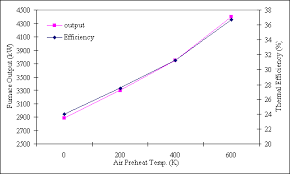The image above represents thermal efficiency of furnace.

To compute for thermal efficiency of furnace, two essential parameters are needed and these parameters are Heat in the Stock (Hs) and Heat in the Fuel for Heating (Hf).

The formula for calculating thermal efficiency of furnace:

Tf = Hs/Hf

Where:

Tf = Thermal Efficiency of Furnace
Hs = Heat in the Stock
Hf = Heat in the Fuel for Heating

Let’s solve an example;
Find the thermal efficiency of furnace when the heat in the stock is 21 and the heat in the fuel for heating is 7.

This implies that;

Hs = Heat in the Stock = 21
Hf = Heat in the Fuel for Heating = 7

Tf = Hs / Hf
Tf = 21 / 7
Tf = 3

Therefore, the thermal efficiency of furnace is 3.

Calculating the Heat in the Stock when the Thermal Efficiency of Furnace and the Heat in the Fuel for Heating is Given.

Hs = Tf x Hf

Where;

Hs = Heat in the Stock
Tf = Thermal Efficiency of Furnace
Hf = Heat in the Fuel for Heating

Let’s solve an example;
Find the heat in the stock when the thermal efficiency of furnace is 10 and the heat in the fuel for heating is 6.

This implies that;

Tf = Thermal Efficiency of Furnace = 10
Hf = Heat in the Fuel for Heating = 6

Hs = Tf x Hf
Hs = 10 x 6
Hs = 60

Therefore, the heat in the stock is 60.